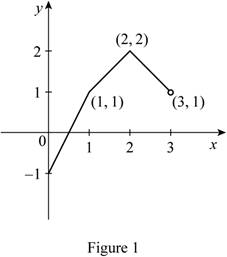# The graph of the function f for the given function f ′ .### Single Variable Calculus: Concepts...

4th Edition
James Stewart
Publisher: Cengage Learning
ISBN: 9781337687805### Single Variable Calculus: Concepts...

4th Edition
James Stewart
Publisher: Cengage Learning
ISBN: 9781337687805

#### Solutions

Chapter 4.8, Problem 39E
To determine

## To sketch: The graph of the function f for the given function f′ .

Expert Solution

### Explanation of Solution

Given data:

Refer to the Figure in the textbook for the given function f .

The function f is continuous on [0,3] and f(0)=1 .

Formula used:

The antiderivative function for the function xn is xn+1n+1+C .

Here, C is the constant.

Calculation:

The function f is the antiderivative function for the function f .

From the given figure in the textbook, the function f is written as follows.

f={2if0x<11if1<x<21if2<x<3

Rewrite the function f as follows.

f={2x0if0x<11x0if1<x<21x0if2<x<3 (1)

Use the antiderivative formula and find the antiderivative function f for the function f in equation (1) as follows.

f={2(x0+10+1)+Cif0x<11(x0+10+1)+Dif1<x<21(x0+10+1)+Eif2<x<3

f={2x+Cif0x<1x+Dif1<x<2x+Eif2<x<3 (2)

Rewrite the function as follows.

f=2x+Cif0x<1 (3)

As f(0)=1 , substitute 0 for x in equation (3),

f(0)=2(0)+Cif0x<1=0+C=C

Rewrite the expression as follows.

C=f(0)

Substitute –1 for f(0) ,

C=1

Substitute –1 for C in equation (3),

f=2x1if0x<1 (4)

From equation (4), the at x=1 , the function f is 1.

f(1)=1 (5)

Rewrite the function in equation (2) as follows.

f=x+Dif1<x<2 (6)

From equation (5), as f(1)=1 , substitute 1 for x in equation (6),

f(1)=1+Dif1<x<2=1+D

Rewrite the expression as follows.

D=f(1)1

Substitute 1 for f(1) ,

D=11=0

Substitute 0 for D in equation (6),

f=x+0if1<x<2

f=xif1<x<2 (7)

From equation (7), the at x=2 , the function f is 2.

f(2)=2 (8)

Rewrite the function in equation (2) as follows.

f=x+Eif2<x<3 (9)

From equation (8), as f(2)=2 , substitute 2 for x in equation (9),

f(2)=2+Eif2<x<3=2+E

Rewrite the expression as follows.

E=f(2)+2

Substitute 2 for f(2) ,

E=2+2=4

Substitute 4 for E in equation (9),

f=x+4if2<x<3 (10)

As the function is continuous on [0,3] , write the function f from equation (4), (7), and (10) as follows.

f={2x1if0x<1xif1<x<2x+4if2x<3 (11)

Sketch the graph of the function f from equation (11) as shown in Figure 1.Thus, the graph of the function f for the given function f is sketched.

### Have a homework question?

Subscribe to bartleby learn! Ask subject matter experts 30 homework questions each month. Plus, you’ll have access to millions of step-by-step textbook answers!